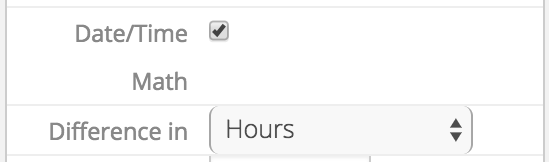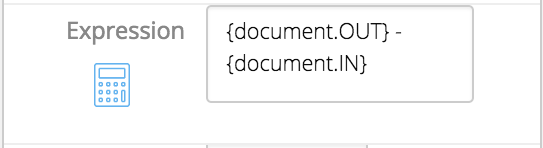# Complex Document Expressions

#### Drive Time Expressions

These expressions allow you to get the travel time in minutes between two valid locations. Both locations must be geocoded (have a valid latitude and longitude) in order for this to work.  The expression starts with driveTime, then has both locations. These locations are pulled from location objects within Field Squared via standard string expressions.

This example would get how long it would take to drive from the parent task to the first asset linked to that task.

#### Document Traversal

These expressions allow you to populate a document control with data from a sibling document. The two documents MUST be on the same object. The document is found by the type (the binding of the form). If there are multiple documents of that type, it will simply use the first created. The binding is that of the control in the document. As with all document expressions, ‘data.’ is NOT included. These expressions work on all Field Squared objects.

This will go to the first document with the binding LABOR on the parent task and populate the doc control with value of the control with the binding PRICE.

#### From Document to Parent Object

These expressions populate a custom field on the parent object with a value pulled from a document. These documents must be on the parent object it's populating to. The document is found by type (the binding of the form). If there are multiple documents of that type, it will simply use the first created. The binding is that of the custom field. As with all document expressions, ‘data.’ is NOT included. These expressions work on all Field Squared objects.

This will go to the first document of binding TOTALFRM on the parent task and populate the custom field with value of the control with the binding SUBTOTAL.

#### Populate Details Tables from Child Tasks

A details table can be filled with data from child tasks. Each row of the details table will be the associated with one child task. The mappings within the .Select() show where the data will be stored. The first value is where the data is coming from, and the value after the arrow is the binding on the details table where that value will be stored.

This would be on a contact, and would go to each child task. The binding on the details table column DATE will be populated by the custom field (thus the ‘.data’) with the binding TASKDATE. The details table column CREATED would be populated by the task date created. Since it is a core field, it is not prefaced by data.

#### Populate Details Tables from Child Assets

A details table can be filled with data from child assets. Each row of the details table will be the associated with one child asset. The first half of the expression narrows down the type of asset you are looking for. From there, we .Select() the mappings for the details table. The first value is where the data is coming from, and the value after the arrow is the binding on the details table where that value will be stored.

{asset.children.Where(type.key == 'Park').Select(name -> ASSETNAME, data.REGION -> ASSETREGION)}

This example would go to each child asset of the type Park. The binding on the details table column ASSETREGION will be populated by the custom field (thus the ‘.data’) with the binding REGION. The details table column ASSETNAME would be populated by the asset name. Since Name is a core field, it is not prefaced by data.

#### Date Time Math

The time between two dates/times can be calculated on a math control. The expression utilizes subtraction, taking the later date from the earlier one.

In order to specify that we want to perform date math, select the option Date/Time Math in the property controls. From there,  you can select in what format you want the difference. The options are minutes, hours and days.This will show the time difference between two controls in the same document, with the bindings OUT and IN.#### Get Status Data

These expressions allow you to get data from the last instance of a status change. This can be pulled into a document on a task, or from the first task on a Field Squared object. The expression is written by accessing the task, then specifying the status. In order to get the date/time from that status, .Date is added at the end.

In this example, the document is on a task, and we want the date/time when the status was changed to "Driving".

In this example, the document is on a contact, and we want the date/time when the status was changed to "Complete" on the contact's first related task.

#### Document Operations with Multiple Conditionals

These expressions find a value on a document when multiple conditions are met. Standard fields are found with expressions like:

type=='FRM123'

and document controls are found with expressions like:

REGION=='South'

combining these looks like:

(type=='FRM123' && REGION == 'South')

This would get the document value for the control with the binding CONTACT on the first document where the type is ‘FRM123’ and it has a control REGION with the value ‘South’:

{asset.documents.Where(type=='FRM123' && REGION == 'South').CONTACT}

#### Document Count Operations

Returns the count of documents on the same parent object that meet the conditions within the expression.

This expression would return the number of documents on the asset that are of the type 'FRM123':

{asset.documents.Where(type=='FRM123').Count()}

This example would return the number of documents on the asset of any type where the doc control with the binding REGION equals 'South':

{asset.documents.Where(REGION == 'South').Count()}

This would return the number of documents on the asset of the type 'FRM123' where the doc control with the binding REGION equals 'South':

{asset.documents.Where(type=='FRM123' && REGION == 'South').CONTACT}

#### Document Sum Operations

Gets the sum from a binding where documents on the same parent object meet the conditions within the expression.

This example would return the total of all doc controls with the binding OCCURRENCES on documents on the asset that are of the type 'FRM123':

{asset.documents.Where(type=='FRM123').Sum('OCCURRENCES')}

This expression would return the total of all doc controls with the binding OCCURRENCES on documents on the asset that have a doc control with the binding 'REGION' that equals 'South':

{asset.documents.Where(REGION == 'South').Sum('OCCURRENCES')}

This expression would return the total of all doc controls with the binding OCCURRENCES on documents  of the type 'FRM123' on the asset that have a doc control with the binding 'REGION' that equals 'South':

{asset.documents.Where(type=='FRM123' && REGION == 'South').Sum('OCCURRENCES')}